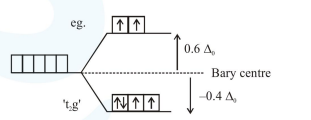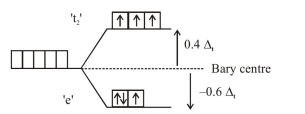# Consider that a d^6 metal ion M^2+ forms a complex with aqua ligands,

Question:

Consider that a $\mathrm{d}^{6}$ metal ion $\left(\mathrm{M}^{2+}\right)$ forms a complex with aqua ligands, and the spin only magnetic moment of the complex is $4.90 \mathrm{BM}$. The geometry and the crystal field stabilization energy of the complex is :

1. tetrahedral and $-1.6 \Delta_{t}+1 \mathrm{P}$

2.  tetrahedral and $-0.6 \Delta_{1}$

3. octahedral and $-1.6 \Delta_{0}$

4. octahedral and $-2.4 \Delta_{0}+2 \mathrm{P}$

Correct Option: , 2

Solution:

If spin only magnetic moment of the complex is $4.90 \mathrm{BM}$, it means number of unpaired electrons should be 4 .

(A) In octahedral complex: $\left[\mathrm{M}\left(\mathrm{H}_{2} \mathrm{O}\right)_{6}\right]^{2+}$

$d^{6}$\begin{aligned} \text { C.F.S.E. } &=\left(-0.4 \Delta_{0}\right) \times 4+\left(+0.6 \Delta_{0}\right) \times 2+0 \times \mathrm{P} \\ &=-0.4 \Delta_{0} \end{aligned}\begin{aligned} \text { C.F.S.E. } &=\left(-0.6 \Delta_{t}\right) \times 3+\left(+0.4 \Delta_{t}\right) \times 3+0 \times \mathrm{P} \\ &=-0.6 \Delta_{t} \end{aligned}